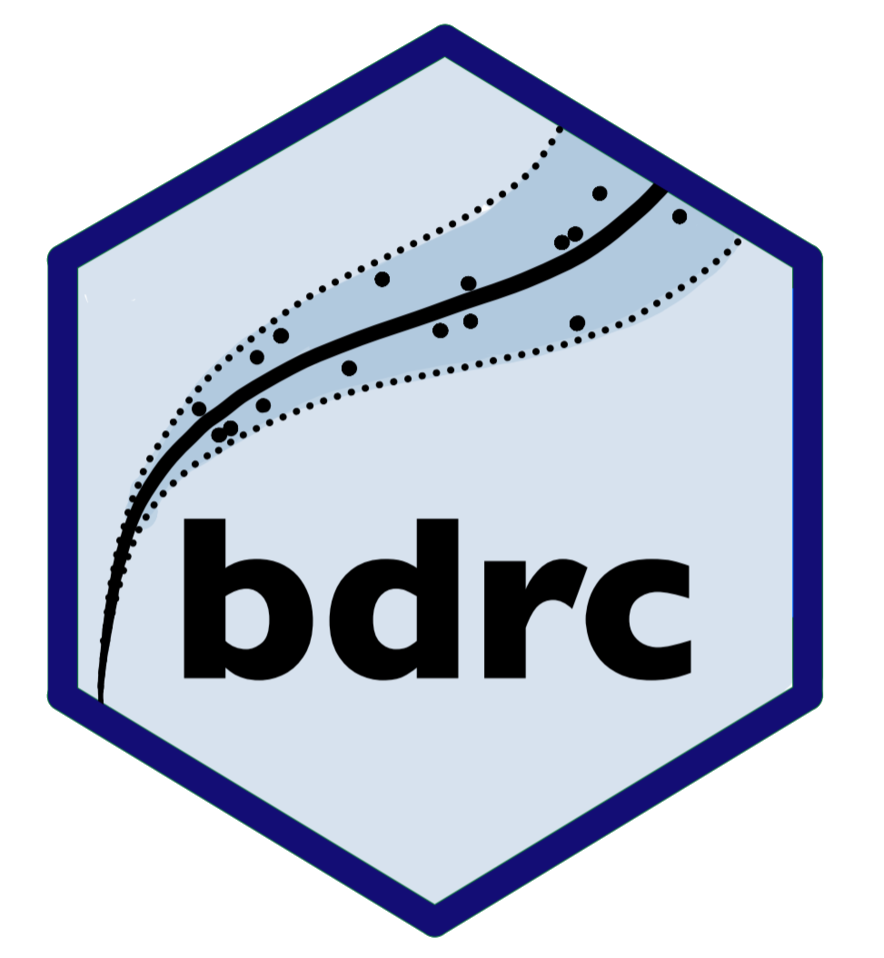## bdrc - Bayesian Discharge Rating CurvesThis software package fits a discharge rating curve based on the power-law and the generalized power-law from data on paired water elevation and discharge measurements in a given river using a Bayesian hierarchical model as described in Hrafnkelsson et al. (2022). Four models are implemented:

`plm0()` - Power-law model with a constant error variance. This is a Bayesian hierarchical implementation of the most commonly used discharge rating curve model in hydrological practice.

`plm()` - Power-law model with error variance that varies with water elevation.

`gplm0()` - Generalized power-law model with a constant error variance. The generalized power-law is introduced in Hrafnkelsson et al. (2022).

`gplm()` - Generalized power-law model with error variance that varies with water elevation. The generalized power-law is introduced in Hrafnkelsson et al. (2022).

## Installation

``````# Install release version from CRAN
install.packages("bdrc")
# Install development version from GitHub
devtools::install_github("sor16/bdrc")``````

## Getting started

It is very simple to fit a discharge rating curve with the bdrc package. All you need are two mandatory input arguments, formula and data. The formula is of the form y~x where y is discharge in m![^3/](https://latex.codecogs.com/png.image?%5Cdpi%7B110%7D&space;%5Cbg_white&space;%5E3%2F “^3/”)s and x is water elevation in m (it is very important that the data is in the correct units). data is a data.frame which must include x and y as column names. As an example, we will use data from the Swedish gauging station Krokfors, which is one of the datasets that come with the package. In this table, the Q column denotes discharge while W denotes water elevation:

``gplm.fit <- gplm(Q~W,krokfors)``

To dig deeper into the functionality of the package and the different ways to visualize a discharge rating curve model for your data, we recommend taking a look at our two vignettes.

Hrafnkelsson, B., Sigurdarson, H., and Gardarsson, S. M. (2022). Generalization of the power-law rating curve using hydrodynamic theory and Bayesian hierarchical modeling, Environmetrics, 33(2):e2711.